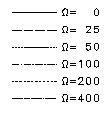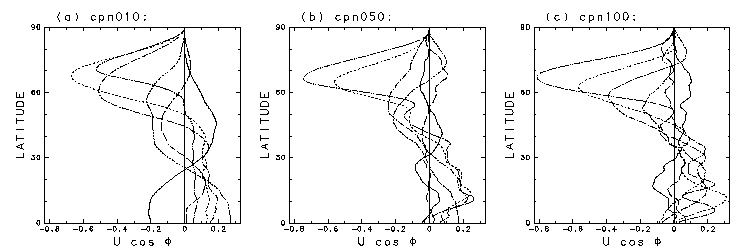4. Results

3.1 Overview of the Time Evolution of the Vorticity Field and the Zonal-Mean Zonal Flow
3.2 Generation of easterly polar vortex
3.3 The Condition Required for the Appearance of the Band Structure

### 3.2 Generation of easterly polar vortexTime evolution was carried out for 18 combinations created from 3 different initial parameters (n0 = 10, 50, and 100) and 6 different rotational angular velocity of sphere (Ω = 0, 25, 50, 100, 200, and 400), and for each combination, calculations were made for 10 different random initial values. The figures below plot the ensemble mean of the final states (t = 5) of the zonal-mean angular momentum of time evolution with the distribution of the southern hemisphere folded over and superimposed on the northern hemisphere. (In other words, we have taken the pseudo ensemble mean for 20 random initial values.)

The other parameters were the same, at γ = 1000 and T341. Note that the distribution curves for each Ω are distinguishable by the line type. (See legend on right.)

 In the case of n0 = 10. In the case of n0 = 50. In the case of n0 = 100In cases of fast rotational angular velocities of the sphere (Ω = 200 and 400), the formation of the polar easterly jets can be confirmed in the ensemble mean for all 3 values of n0. The band structure in the mid-to-low latitudes is not obvious in these plots due to the fact that the latitude at which the bands are generated differ among each run and cancel each other out when the ensemble mean is taken.